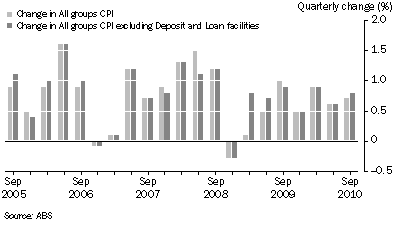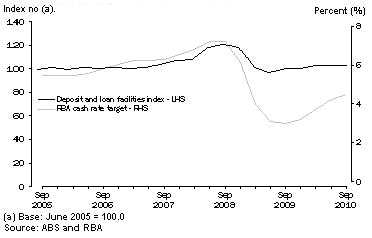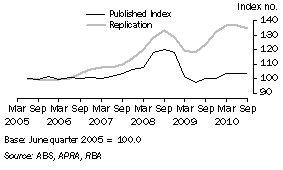6469.0 - Outcome of the 16th Series Australian Consumer Price Index Review, Dec 2010
ARCHIVED ISSUE Released at 11:30 AM (CANBERRA TIME) 06/12/2010  First Issue
Page tools:Print All

APPENDIX 6 EVALUATION OF THE DEPOSIT AND LOAN FACILITIES INDEX

INTRODUCTION

1. This appendix provides a summary of the methodology used to calculate the indirect fees component of the Deposit and loan facilities index and provides details of ABS analysis of some of the methodological concerns raised by users. For data confidentiality reasons, the ABS is unable to present detailed analysis. Where possible, qualitative descriptions have been provided.

SUMMARY OF CURRENT METHODOLOGY FOR INDIRECT FEES

2. To calculate the indirect banking service charge in the CPI, monthly balance and interest flow data are obtained from selected financial institutions for each of their consumer products and in aggregate. A separate reference rate of interest is calculated for each institution as the mid-point of weighted average borrowing and lending rates. For each institution, a sample of products is selected to represent each of the major product categories. The specific products selected from each group (e.g. the sampled home loan product) are assigned a weight to represent the entire product group (e.g. housing loans). The product yield for each sampled product is determined by dividing the annualised interest by the product balance. The interest rate is calculated from the difference between the product yield and the reference rate (for deposit accounts the interest margin is the reference rate less the product yield, for loan accounts it is the product yield less the reference rate). Because percentages (such as margin rates) are not prices, the latest period margin rates have to be applied to some monetary amount in order to compute the current period prices (the dollar value of the margins). Balances on a series of sampled accounts are used for this purpose. To preserve the quantities underpinning the values of the account balances in the base period, the balances used to derive the dollar values of the margins are updated each period using a four-quarter moving average of the All groups CPI. The indirect component of the Deposit and loan facilities index is calculated by weighting the indexes for the sampled products according to the weight of the product group. To minimise the effect of any short-term accounting anomalies, such as posting effects and adjustments of various types, the ABS constructs three-month moving averages of the average balances and interest flows and derives the required interest rates, reference rates and margin rates from the smoothed data.

Table 1 - Summary of Deposit and loan methodology calculation

 Calculate average interest rate (yield) on deposits (and loans) for each sampled institution YD=(Sum of interest on all deposit products)/(Average balance on all deposit products) YD - yield on deposits Yl - yield on loans Calculate mid-point reference rate for each sampled institution RR= (YD+YL )/2 RR - reference rate Calculate average interest rate (yield) on each product Yi= Ii/Bi Ii - interest on product i Bi - average balance on product i Yi - yield on product i Calculate interest rate margin on each sampled product from difference between reference rate and product yield Mi = Yi - RR (for loans) Mi = RR - Yi (for deposits)Derive constant balance by indexing base period balance B*i .CPIt/CPI0 CPIt - lagged four quarter moving average of the CPI Calculate dollar value of interest rate margin (price) by applying interest rate margin to indexed base period balance Pi = Mi.B*i .CPIt/CPI0All calculations are performed on three month moving averages of data, lagged by one month.

3. The weight of the indirect fee component of the Deposit and loan facilities index is calculated in a manner consistent with the methodology, as follows:

a) Margin and other fee calculation for sampled financial institutions, by product.

The percentage margin on each product is calculated as described above and the dollar value of the margin computed by applying the current period margin rate to the balances of the sampled accounts.

For all those products identified as being consumer products (as distinct from those used by businesses), the total receipts from households are calculated by summing the margins.

b) Scaling up to all domestic deposit-taking institutions

The aggregate ratio of these receipts to total balances for the sampled institutions is applied to aggregate balances for all deposit taking institutions to derive a national estimate i.e. scaling up sampled banks to all deposit-taking institutions. Banks, building societies and credit unions comprise the definition of deposit taking institutions.

c) Regional (state) distribution

For the regional distribution specialised state financial balance data is used.

d) Scaling down to the eight capital cities

The capital city level estimates were imputed by reference to aggregate data from the household expenditure survey.

4. A fuller description of the methodology, including the methodology used to calculate the direct fees component, can be found in the Appendix (p.34-36) of the June quarter 2008 issue of the CPI or Appendix 4 of Information Paper: Issues to be considered during the 16th series Australian Consumer Price Index (CPI) review (cat. no. 6468.0).

5. The impact of the Deposit and loan facilities index on the All groups CPI is shown in Graph 1, which compares the published figures for changes in the All groups CPI with changes in the All groups CPI excluding Deposit and loan facilities. The largest difference is observable in the March quarter 2009.
Graph 1 - Changes in the All groups CPI and the All groups CPI excluding Deposit and loan facilitiesVOLATILITY AND CORRELATION WITH THE RBA CASH RATE TARGET

6. The volatility of the Deposit and loan facilities index and its correlation with the RBA cash rate target is evident from Graph 2. Quarterly movements as large as an increase of 9.5% in the June quarter 2008 (in part the result of a correction) and a decrease of 14.1% in the March quarter 2009 have been observed. The correlation coefficient between the change in the Deposit and loan facilities index and the change in the RBA cash rate in the previous quarter is 0.9.
Graph 2 - CPI Deposit and loan facilities index and the RBA cash rate targetSENSITIVITY TO INTEREST RATE CHANGE AND DATA ERRORS

7. If symmetric increases of 1% are applied to all product yields, the reference rate increases by the same amount (1%) and the spread between deposit and loan yields remains constant. The interest rate increases cancel out in the calculation, leaving the percentage margins on all products unchanged. In this case, the ABS methodology delivers an index that only changes with the change in the lagged four-term moving average of the CPI (due to the indexation of base period balances).

8. If yields on loans increase more than yields on deposits, the spread between average interest rates will widen, which (in most cases) leads to an increase in the index. The margins on most products increase, leading to an increase in the index above the change in the lagged four-term moving average of the CPI. This demonstrates that, in general, the methodology will deliver an increase in the index (above the balance indexation) if yields on loans increase more than yields on deposits. This is appropriate if the spread between deposit and loan yields are widening purely as a result of bank behaviour. If however, banks are increasing loan interest rates to compensate for increasing costs of whole sale funding that are not captured by the ABS reference rate, a price increase in the indirect fees will be inappropriately reported. To take increases in costs of wholesale funding into account would involve a major change to the current model.

9. Sensitivity analysis showed that errors on products with large balances, small margins or large weights have the greatest impact on the index. This highlights the need for extremely accurate data. The impact of aggregation on the index, particularly in unstable economic times, emphasises the requirement that estimates are based on the detailed data for individual products. The differences between sampled and census indexes also imply that a more reliable index will be obtained if data is available on all products. To produce an index robust under all economic conditions the ABS is negotiating further with data providers.

TREATMENT OF FIXED RATE PRODUCTS

10. The impact of fixed rate products on the Deposit and loan facilities index was assessed by comparing the index with one calculated by excluding fixed rate products. The two indexes showed remarkably different behaviour in times of interest rate volatility, as fixed rate product yields moved very differently from the reference rate. Alternative treatments of fixed rate products such as using a reference rate matched to the product maturity reduced both the volatility and impact on the index of fixed rate products. Treatment of fixed rate products are a subject of international debate in which the ABS is involved and it would be premature to reach a conclusion prior to consensus of the international community.

SAMPLING STRATEGY

11. The difference between sampling a single product, three dominant products, and all products within each product group (a census of products) was examined. The three sampling strategies led to minor differences in the index, which indicates that the current strategy may produce an index that is not representative of all products. To avoid these issues the ABS is aiming to produce output using a census index containing all products.

FREQUENCY OF LOWER LEVEL WEIGHT UPDATING

12. Low level weight updating (below the expenditure class level) is common practice in the CPI. A superior result is obtained if the relative weights of product groups are updated annually. The ABS recently adopted procedures for routine annual weight updates for the Deposit and loan facilities index.

ALTERNATIVE REFERENCE RATES

13. Some users were concerned about the use of a mid-point, reference rate. To address these concerns the index was recalculated using both the interbank rate (as recommended by the System of National Accounts) and a single mid-point reference rate for all institutions. The results were strongly dependent on the choice of reference rate. During the time period studied, the index using the interbank rate was extremely volatile compared with current practice. This is related to the relative impacts of divergence between the loan interest rates and the interbank rate. The current choice of reference rate delivers the least volatile alternative.

FORECASTING, INDEPENDENT ESTIMATION, DETAIL AND AGGREGATION

14. Graph 3 shows a comparison between the published series and the result obtained when the ABS methodology is applied to publicly available data. Average nominal (rather than transactional) interest rates are available from the RBA website by product group (although these are not split by institution). End of month balance data by financial institution is available from the Australian Prudential Regulation Authority (APRA) website. These monthly data are aggregated for total household deposits and household loans are aggregated by credit cards, housing loans and other loans. The two series show both clear similarities and differences due to the reasons discussed in Chapter 4. One major source of difference is the level of aggregation.
Graph 3 - CPI Deposit and loan facilities index and replication using publicly available data15. The CPI is a fixed basket index, i.e. the quantities are fixed in a base period and are re-priced each quarter. The current Deposit and loan facilities index methodology achieves this by repricing indirect fees on sampled products (the finest level of detail available). The publicly available data, however, is aggregated to a higher level. This caused difficulty for comparison as price changes calculated on aggregated datasets are subject to bias from compositional shift. For example, the measured average interest margin for a grouping of products that combines both home loan products and credit cards may vary from period to period depending on the relative balances on home loans and credit cards rather than any change in individual margins. If products are aggregated such that homogenous items are grouped, e.g. all home loans products, the effect may be minor. When dealing with heterogenous groups with very dissimilar prices, e.g. across product groups such as home loans and credit cards, compositional shift will be much more significant.

16. The impact of compositional change was investigated by comparing a) a completely disaggregated dataset with the same dataset in which: b) only fixed and variable rate loans were aggregated, c) data was aggregated to total household deposits and household loans was aggregated by credit cards, housing loans and other loans (as in our publicly available dataset), and d) all deposit data was aggregated as was all loan data. The differences between these series indicated that an accurate index can only be assured if data are disaggregated to the finest level of detail.